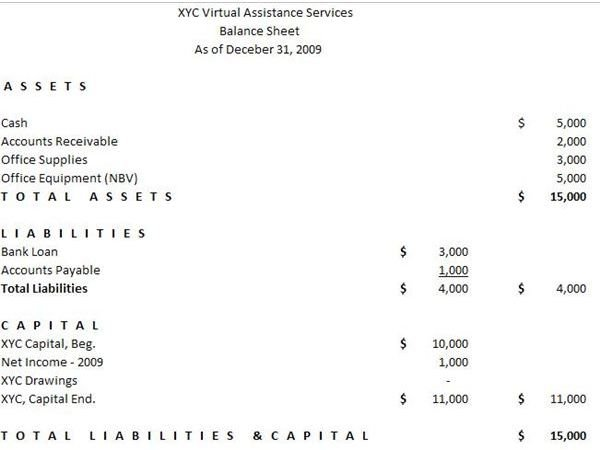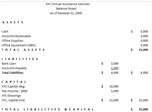# What is the Fundamental Accounting Equation?

## Fundamental Accounting Equation and Its Significance in a Balance SheetUnderstand that the query "What is the fundamental accounting equation?" is answerable by explanations about the basic accounting equation. Both refers to the equation Assets = Liabilities + Capital, which is the same format for balance sheet presentation.

The balance sheet is a report containing a summary of how much the business owns in terms of assets. In relation to this, the assets will have a breakdown on how much of it will be used to pay off liabilities and how much of the assets is comprised by its capital. Each of these three balance sheet components have summary totals and can be simply summarized into the fundamental accounting equation.

This is why the balance sheet is considered as an all important tool by management and by external users, in order to get a quick look as to what the business is made of.

To visualize, click on the image of a sample balance sheet to get a larger view.

## Transposing the Fundamental Accounting Equation and What it Tells

To give the reader an idea on how management and external users utilize the fundamental accounting equation, a further analysis will be made by transposing the fundamental accounting equation, giving emphasis to each balance sheet component. Starting off with the basic equation, we will be able to learn from our sample balance sheet that:

• Assets (\$15,000) = Liabilities (\$4,000) + Capital (\$ 11,000)

Based on the sample balance sheet's basic accounting equation, management and external users will readily perceive that not all of the business assets are free or unencumbered or wholly constituted by the capital investment. At first glance, others may even have the immediate impression that the business does not wholly own most of its assets. Otherwise, the equation could have been as simple as Assets = Capital.

As proof of the balance sheet totals and, if emphasis were to be given to the liability component, the fundamental accounting equation can be transposed as:

• Liabilities (\$4,000) = Assets (\$15,000) – Capital (\$11,000)

Through this form of equation presentation, it can be seen that the total liabilities is equivalent to the excess of the assets over its capital investment. Asset accounts are normally derived from the capital infused and from the income gained in investing said capital. The transposed form of the fundamental accounting equation shows that the increase in assets was not derived from capital but from borrowings or liabilities.The total of the liabilities is equal to that part of the assets that was not derived from capital resources.In analyzing the compositions of our sample balance sheet, further explanations are provided about the total liabilities.

Understand that in the event that the business is liquidated, \$ 4,000 of the cash included as assets will be used to payoff the liabilities. This leaves the owner a net asset value of only \$11,000 available for liquidation.

Again, the fundamental accounting equation can be transposed to determine how much is the present capital or equity of the owner as far as the business is concerned, via this equation:

• Capital (\$11,000) = Assets (\$15,000) – Liabilities (\$4,000)

The basic accounting equation above readily shows that the current value of the owner’s equity is \$11,000. Reference will then be made as to how much is the original investment, by referring to the account captioned as "Capital, Beginning" of \$10,000. The \$1,000 increase represents the net income for the whole year, which could be considered as slow .It appears that the business earns an average of \$83.33 only, as monthly income (S 1,000 / 12 months).

Consider the total liabilities of the business and the annual income that was earned — obviously, the amount will not even compensate for the amount of interest being paid for the loan. Thus, it could be largely surmised that the business income is being burdened by the interest paid on the loan.

As we go deeper into our analysis of the fundamental accounting equation, we will also be looking into the sub-components of the total assets. You will note that the asset total includes an accounts receivable balance of \$2,000. This is another negative indication in our sample balance sheet, since it could be perceived that the \$1,000 net income is still subject to collection.

As a summary of this case study, an in-depth analysis of the basic accounting equation will disclose that the owner encounters difficulty in collecting actual money earned for services already rendered. As a result, the owner has to resort to borrowing instead of relying on income generated by the business. If the \$ 2,000 receivable was collected during the year in operation, that and the money used for paying the interest on the loan, could have lessened the need for outsourcing business funds.

The above analysis and the different transpositions presented, provide the answers to the query "What is the fundamental accounting equation?'

It is a summarized form of presenting the true picture of the business by showing the relation of its assets to its liabilities and capital. It is important, therefore, that the balance sheet components are kept balanced, since they represent the basic accounting equation.

## Reference:

Sample balance sheet and explanations were created for this article by the author.